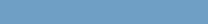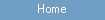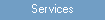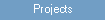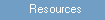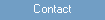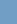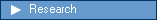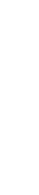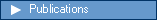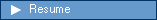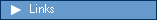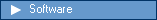Research An Optimal Adaptive Power System Stabilizer (1997) An optimal control algorithm with adaptive system parameters and state variables estimation is presented. The optimal control is calculated by solving the algebraic Riccati equation of the linearized closed loop system model obtained by using an adaptive control strategy. For real-time application feedback control is achieved by recalculating the control sequence each sampling period. An application of the algorithm a power system stabilizer is demonstrated in real-time environment. H Infinity Model Predictive Control - Theory and Application (2005) Future industrial systems will require control systems to be more reliable, autonomous, robust, and yet efficient. The emphasis of this thesis is to introduce a robust control algorithm definition that addresses the needs of future industrial environments. The proposed controller is based on an adaptive concept with a two-step approach. In step one, the system model is identified in a closed-loop by a robust technique. In step two, the obtained system model from step one is used to formulate a robust controller.